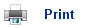# Entropy and the Second Law of Thermodynamics

## Entropy of the Surroundings

• Why does water freeze at temperatures below 0oC?
• Water has a greater entropy than ice and so entropy favours melting.
• But ice has a lower energy than water and so energy favours freezing.
• It is possible to predict what will happen by taking into account the entropy of the surroundings, in addition to the energy of the system.
• The following reaction shows the change in state from water to ice:• Freezing is an exothermic process; energy is lost from the water and dissipated to the surroundings.
• Therefore, as the surroundings get hotter, they are gaining more energy and thus the entropy of the surroundings is increasing.
• The amount by which the entropy of the surroundings has increased can be determined using the following principle: the entropy of the surroundings increases by an amount equal to the heat energy they gain divided by the temperature at which it happens, therefore:• This relationship allows us to make a prediction about the entropy of the surroundings of a chemical process, whatever they are (even the whole universe!), using the measurements we can make on the chemical system.
• The total entropy change can then be used to predict whether a reaction is feasible or not at a given temperature.
• The total entropy is equal to:• Using this equation for the freezing of water at -10oC for example:• The overall entropy increases, and so the reaction is feasible (in thermodynamic terms, the reaction would be described as being spontaneous; however, this does not in any way describe how fast the reaction occurs).
• The feasibility of the reaction can then be determined at 10oC: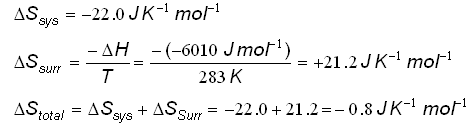• At the higher temperature, the reaction is not feasible as the entropy of the reaction is decreasing. However, ice will melt at this temperature, as the entropy increases :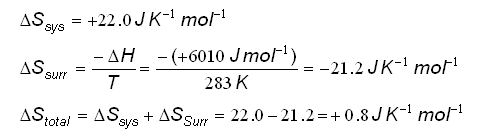• Both the processes of ice melting at 10oC and water freezing at -10oC are spontaneous reactions; they can occur. Both these reactions occur as they result in an increase in the total entropy.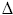Stotal is positive for a spontaneous reaction

• This is part of the Second Law of Thermodynamics and it is very important as it allows us to predict whether something can happen or not.
• Even though a reaction can be spontaneous, it is not certainty that the reaction will occur, as the activation energy may be too high for the reaction.
• The second law of thermodynamics can be used to explain the following:1. The reaction will occur, as in an exothermic reactionH is negative, and if the entropy increases, thenS is positive, so: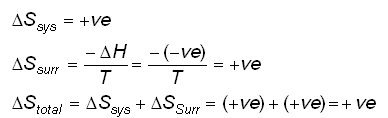Total entropy change is positive, so reaction is feasible.
2. The reaction can never occur, asH is positive andS is negative: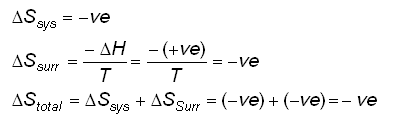The total entropy change is negative and so the reaction cannot occur.
3. The reaction can occur depending on the conditions: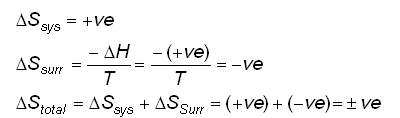As the temperature increases,Ssurr increases (becomes less negative), therefore, the higher the temperature, the more likely it is for the reaction to occur. This ties in with La Chatelier’s principle- an increase in the temperature of an endothermic reaction pushes the position of equilibrium to the product side.
4. The reaction can occur depending on the conditions: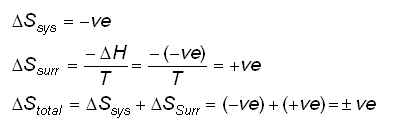As the temperature decreases,Ssurr increases, therefore, the lower the temperature, the more likely it is for the reaction to occur.

## Summary

• When changes occur in a chemical reaction, they are nearly always accompanied by changes in the surroundings.
• To predict whether or not a change will take place, we need to take account of the entropy changes in the system and its surroundings.• For a change to be spontaneous, the total entropy must increase.
• The Second Law of Thermodynamics states that for a spontaneous reactionStotal > 0.

## Using the Second Law of Thermodynamics- Saltwater freezing

• Have you ever wondered why salt water freezes at a lower temperature than pure water? Why icebergs are present in sea water and why putting salt on the road in winter prevents ice from forming?
• This is all related to the process: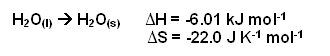• When seawater freezes, pure ice is produced; the energy released will be the same as when molecules from pure water fit together to form a lattice in an ice crystal.
•H will still be -6.01 kJ mol-1 when seawater freezes.
• However,S for the overall system is different.
• The entropy of the salt solution is greater than the entropy of pure water; the Na+ and Cl- ions are spread out in the solution.
• When salt water freezes, theS is more negative, due to the fact that the salt water is more disordered, and so it becomes more ordered when the water frezzes.
• Therefore, theSsys for salt water freezing is less than -22.0 J K-1.
• If theSsys for salt water freezing is lower, then theSsurr has to be more positive than +22.0 kJ mol-1 in order for the reaction to be spontaneous.
• From the following equation: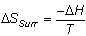Ssurr can only be greater than +22.0 kJ mol-1 if we divide -H by a smaller value of T, so the freezing of salt water takes place at a temperature lower than 0oC.

## Why limestone doesn’t dissolve

• At high temperatures, calcium carbonate can thermally decompose to form calcium oxide and carbon dioxide:• At normal temperatures on Earth, this process does not occur; this, once again, is related to the Second Law of Thermodynamics.
• The following shows the entropy change at 25oC (298K):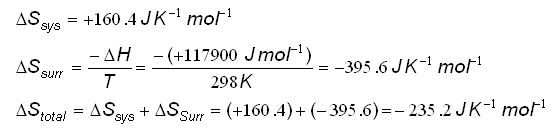• The total entropy change for this reaction is negative; therefore it is not spontaneous.
• At 1273K, the entropy change is as follows: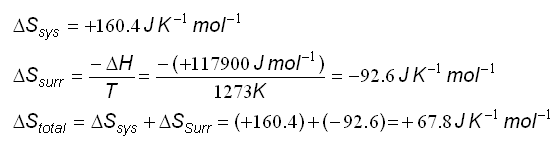• At the higher temperature, theSsurr is less negative and so theStotal becomes positive, meaning that the reaction is feasible at the higher temperature.

## Dissolving and Crystallisation

• Why should salt dissolve to produce a solution (like sea water), but then crystallise from solution in the salt works when most of the water has evaporated?
• The process of the salt dissolving is:• The entropy change is as follows:• At 298K, the entropy change is +26.1 J K-1 mol-1, so salt can dissolve to form a solution at 25oC.
• If the solution is more concentrated, then theSsys value will change substantially:
• There is a smaller volume of water for the ions to be dispersed in, thus there will be fewer possible arrangements of their positions.
• The water molecules will hydrate the ions, and will therefore become more organised, rather than randomised in the liquid.
• Therefore, the organised water molecules will make up a greater proportion of the water present.
• Both of these result in theSsys decreasing for the more concentrated solution.
• Eventually theSsys becomes less than 13.1 J K-1 mol-1, which leads to theStotal becoming negative, and so dissolving becomes unfavourable and crystals form from the solution.

## Useful books for revision

Revise A2 Chemistry for Salters (OCR A Level Chemistry B)Salters (OCR) Revise A2 ChemistryHome